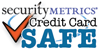item# 2140-1500

# 30" White Elastic Neck Cord with metal barbed ends for 2-hole ID Cards

SIZE
30" long ( metric: 762mm )
THICKNESS
approximately 1/16"
QUANTITY
each
MATERIAL
elastic
CONNECTOR 2 NPS barbed ends
COLOR
White
QUANTITY DISCOUNT PRICING

1 to 9 = \$3.95 / each

10 to 24 = \$1.37 / each

25 to 49 = \$0.62 / each

50 to 99 = \$0.38 / each

100 to 249 = \$0.26 / each

250 to 499 = \$0.20 / each

500 to 999 = \$0.16 / each

1000 to 2499 = \$0.15 / each

2500 to 9999 = \$0.13 / each

Quantity: